# How to get multiple values into one cell.

###### Thursday, December 17, 2015

Tags: business objects, concat, sap, tables, web intelligence

Here I would like to describe how to get multiple values from rows into one single cell as a comma separated list in 4 steps with 4 variables

I will take standard eFashion universe as an example. Let's work on LINES and CATEGORY objects. Here is the raw list from the universe for LINES in (Dresses, Jackets, Leather):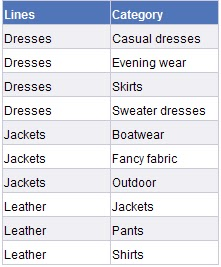And what we want here is to be able to list categories as a comma separated list next to each line. So this will be our desired goal: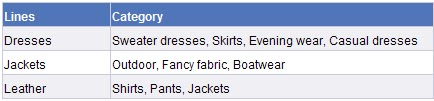STEP #1 (find the maximum category, with respect to each LINE)

Create a variable [Max Category]

Max([Category]) In ([Lines])

STEP #2 (concatenate the category values with its previous row value)

Create another variable [Concat Category]

[Category] +", "+ Previous(Self)

With these variables, it would look like this: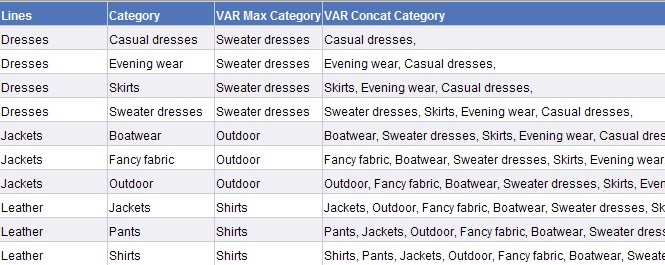STEP #3 (get those rows of [Concat Category] where the value of the category is maximum for that specific LINE)

Create yet another variable [Max Concat Category]

[Concat Category] Where ([Category]=[Max Category])

Therefore we have: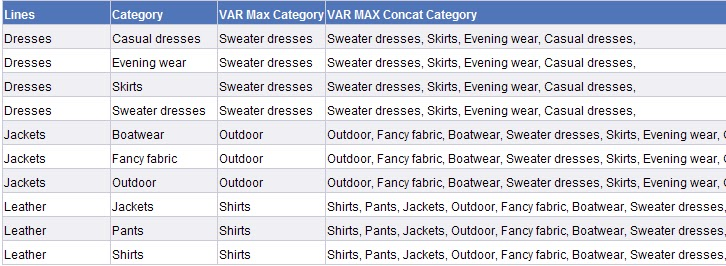If we remove or hide the columns between it would actually look like this: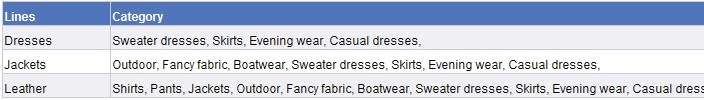As you can see, the lists are cumulative and a comma at the end. So our next step will be to eliminate these.

STEP #4 (display those categories that belong to their Lines, against each of their respective Lines)

Create a final variable [Category]

If(IsNull(Previous([Max Concat Category]));Substr([Max Concat Category];1;Length([Max Concat Category])-2);Substr([Max Concat Category];1;Pos([Max Concat Category];Previous([Max Concat Category]))-3))

Basically, this formula checks if the previous value of [Max Concat Category] is NULL.

If so, then it just displays [Max Concat Category]

In the above image, this scenario occurs for LINE = Dresses. There is no value for [Max Concat Category] prior to LINE = Dresses and soit is NULL.

Otherwise previous row value of [Max Concat Category] is removed from the present row value of [Max Concat Category]

This scenario occurs for LINE = Jackets and LINE = Leather (and all the rows that may occur below). This is done with the help of POS (for finding the position where the previous value of [Max Concat Category] starts), and SUBSTR functions to get the desired parts of the text.

So this would reach us our desired outcome: